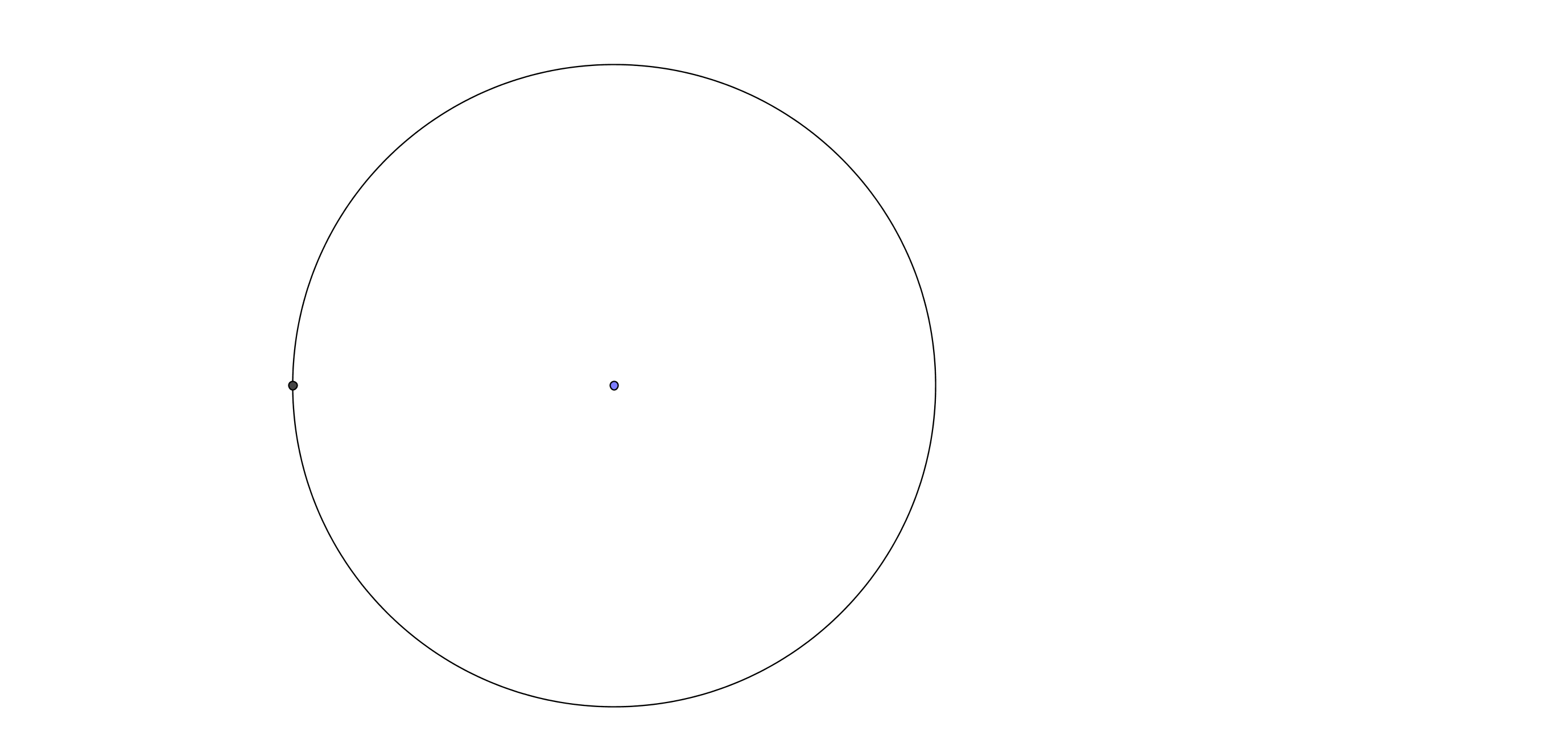# GEOM 1 | Lesson 1 | Watch (Area of Circles)# Area of Circles

Area of a circle:

Draw a circle either freehand or go to GeoGebra to create a circle, cut the circle into two semicircles. How could you make the semi circles form a parallelogram? A rectangle?  What if you were to divide the circle in quarters or eighths?Think about how the area of circle is related to the area of a parallelogram. See the video below for a complete explanation.

Watch the video below.  How did your thinking compare to the video?   Return to this page by using the back arrow.

Area of a circle, how to get the formula.

You can also find the circumference of the circle by using the formula 2πr so you can also find the length of a part of a circle called an arc.  Watch the following video to review finding the length of an arc.

Arc Length